# Exercise E10.4 Circles NCERT Solutions Class 9

## Chapter 10 Ex.10.4 Question 1

Two circles of radii $$5 \rm{cm}$$ and $$3 \rm{cm}$$ intersect at two points and the distance between their centers is $$4 \rm{cm}.$$ Find the length of the common chord.

### Solution

What is known?

Radii of two circles and distance between the centers of the circles.

What is unknown?

Length of common chord.

Reasoning:

Perpendicular bisector of the common chord passes through the centers of both the circles.

Steps: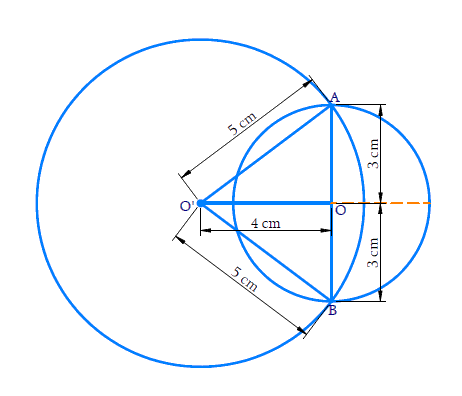Given that the circles intersect at $$2$$ points, so we can draw the above figure.

Let $$AB$$ be the common chord. Let $$O$$ and $$O^\prime$$ be the centres of the circles respectively.

\begin{align} &{O}^{\prime} {A}=5 {\rm{cm}}, {OA}=3 {\rm{cm}},\\ & {OO}^{\prime}=4 {\rm{cm}}\end{align}

Since the radius of the bigger circle is more than the distance between the $$2$$ centres, we can say that the centre of smaller circle lies inside the bigger circle itself.

$${OO}’$$ is the perpendicular bisector of $${AB.}$$

\begin{align} {\rm{So,}} \;{OA}&={OB}=3 \,\rm{cm}\\ {AB}&=3+3=6\, \rm{cm} \end{align}

Length of the common chord is $$6 \,\rm{cm.}$$

It is also evident that common chord is the diameter of the smaller circle.

## Chapter 10 Ex.10.4 Question 2

If two equal chords of a circle intersect within the circle, prove that the segments of one chord are equal to corresponding segments of the other chord.

### Solution

What is known?

Chords are equal. Chords intersect at a point within the circle.

What is unknown?

Proof of corresponding segments of equal chords are equal.

Reasoning:

Equal chords are equidistant from the centre. Using this and Right angled-Hypotenuse-Side (RHS) criteria and Corresponding parts of congruent triangles (CPCT) we prove the statement.

Steps: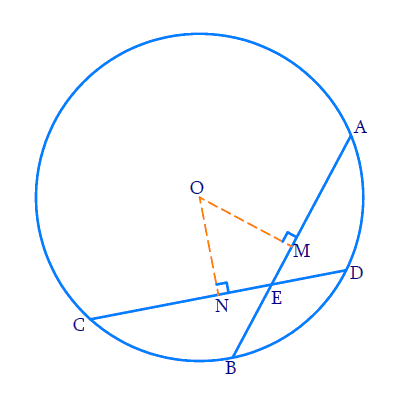Let $${AB}$$ and $${CD}$$ be the $$2$$ equal chords. $${AB = CD.}$$

Let the chords intersect at point $${E}$$. Join $${OE.}$$

To prove \begin{align} {AE = CE \, {\rm{and}} \, BE = DE.} \end{align}

Draw perpendiculars from the center to the chords. Perpendicular bisects the chord $${AB}$$ at $${M}$$ and $${CD}$$ at $${N.}$$

\begin{align} {AM = MB = CN = DN ……(1)} \end{align}

\begin{align} {\rm{In}} \;\Delta {OME}\; {\rm{and}} \;\Delta {ONE}\end{align}

\begin{align}\angle {M}&=\angle {N}=90^{\circ}\\ {OE}&={OE}\\{OM}&={ON} \quad\\\end{align}

Equal chords are equidistant from the centre.

By RHS criteria, $$\Delta {OME}$$ and $$\Delta {ONE}$$ are congruent.

So by $$\text{CPCT}, {ME}={NE} \ldots \ldots(2)$$

We know that: $${CE}={CN}+{NE}$$  and

$${AE}={AM}+{ME}$$

From ($$1$$) and ($$2$$), it is evident $${CE = AE}$$

$${DE}={CD}-{CE}$$ and

$${BE}={AB}-{AE}$$

$${AB}$$ and $${CD}$$ are equal, $${CE}$$ and $${AE}$$ are equal. So $${DE}$$ and $${BE}$$ are also equal.

It is proved corresponding segments of equal chords are equal.

## Chapter 10 Ex.10.4 Question 4

If a line intersects two concentric circles (circles with the same center) with centre $${O}$$ at $$A, B, C$$ and $$D$$, prove that $${AB = CD.}$$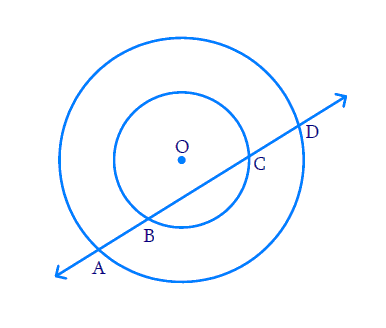### Solution

What is known?

Two concentric circles with centre \begin{align} {O.} \end{align}

What is unknown?

Proof that $${AB = CD}$$

Reasoning:

Perpendicular drawn from the centre of the circle bisects the chord.

Steps: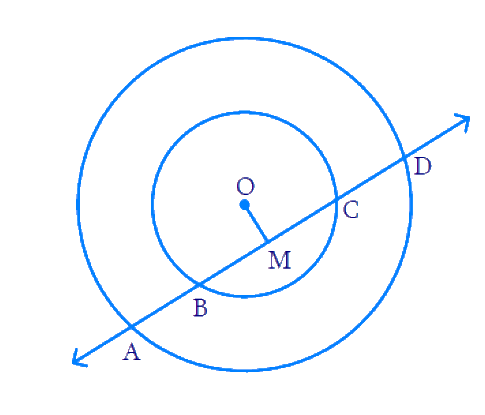Draw a perpendicular from the centre of the circle $${OM}$$ to the line $${AD}$$.

We can see that $${BC}$$ is the chord of the smaller circle and $${AD}$$ is the chord of the bigger circle.

We know that perpendicular drawn from the centre of the circle bisects the chord.

\begin{align}∴ {BM}={MC} \ldots(1)\end{align}

And, $$AM = MD ... (2)$$

Subtracting ($$2$$) from ($$1$$), we obtain

\begin{align}{AM - BM }= {MD} - {MC}\end{align}

\begin{align}∴ {AB = CD}\end{align}

## Chapter 10 Ex.10.4 Question 4

If two equal chords of a circle intersect within the circle, prove that the line joining the point of intersection to the center makes equal angles with the chords.

### Solution

What is known?

Chords are equal. Chords intersect at a point within the circle.

What is unknown?

Line joining the point of intersection to the centre makes equal angles with the chords.

Reasoning:

Equal chords are equidistant from the centre. Using this and Right angled- Hypotenuse-Side (RHS) criteria and Corresponding parts of congruent triangles (CPCT) we prove the statement.

Steps: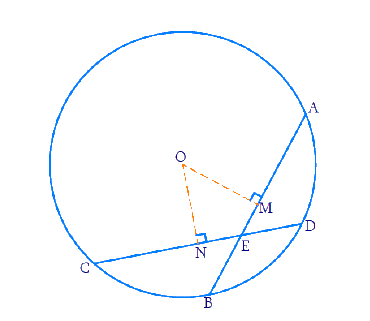Let $${AB}$$ and $${CD}$$ be the $$2$$ equal chords. $${AB = CD.}$$

Let the chords intersect at point $${E}$$. Join $${OE.}$$

Draw perpendiculars from the centre to the chords. Perpendicular bisects the chord $$AB$$ at $$M$$ and $$CD$$ at $$N$$.

To prove: $$\angle {OEN}=\angle {OEM}$$

$${\rm{In}} \;\Delta {OME}\; {\rm{and}} \;\Delta {ONE}$$ \begin{align} \angle {M}&=\angle {N}=90^{\circ}\\ {OE}&={OE}\\ {OM}&={ON} \quad \\\end{align}

( Equal chords are equidistant from the centre.)

By RHS criteria, $$\Delta {OME}$$ and $$\Delta {ONE}$$ are congruent.

$$\text{So by CPCT, }\angle {OEN}=\angle {OEM}$$

Hence proved that line joining the point of intersection of $$2$$ equal chords to the centre makes equal angles with the chords.

## Chapter 10 Ex.10.4 Question 5

Three girls Reshma, Salma and Mandip are playing a game by standing on a circle of radius $$5\rm\,m$$ drawn in a park. Reshma throws a ball to Salma, Salma to Mandip, Mandip to Reshma. If the distance between Reshma and Salma and between Salma and Mandip is $$6\,\rm m$$ each, what is the distance between Reshma and Mandip?

### Solution

What is known?

Three players are standing in a circle. Distance between two pairs is given. Radius of circle is given.

What is unknown?

Distance between Reshma and Mandip

Reasoning:

Perpendicular from center to either of the chord bisects the chord.Using this fact and by pythagoras theorem we can find value of $$OA$$. After that we can find area of $$∆ORS$$ by two ways like $$RS$$ as base and $$OA$$ as height or $$OS$$ as base and $$RN$$ as height. From this we will get the value of $$RN$$ and double of value $$RN$$ will will give the distance between Reshma and Mandip.

Steps: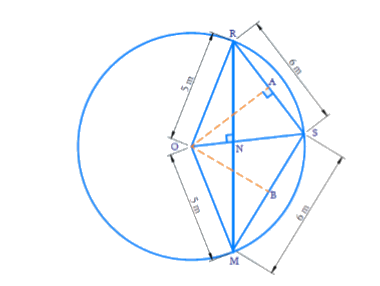Let $$O$$ be the centre of the circle and $$R, M$$ and $$S$$ denote Reshma, Mandip and Salma respectively.

Draw a perpendicular $${OA}$$ to $${RS}$$ from $${O.}$$ Then \begin{align}{RA = AS = 3}\,\rm {m.} \end{align}

Using Pythagoras theorem we get, \begin{align}OA = 4 \text{m.} \end{align}

We can see that quadrilateral $$ORSM$$ takes that shape of a kite.

(Because $$OR = OM$$ and $$RS = SM$$).

We know that the diagonals of a kite are perpendicular and the main diagonal bisects the other diagonal.

$$\angle {RNS}$$ will be $$90^\circ$$ and \begin{align}{RN = NM} \end{align}

$$\text{Area of } ∆ORS$$ \begin{align} &= \frac{1}{2} \times RS \times OA\\ &=\frac{1}{2} \times 6 \times4\\&= 12 \dots(1)\end{align}

Also

$$\text{Area of} ∆ORS$$ \begin{align}& =\frac{1}{2} \times OS \times RN\\&= \frac{1}{2} \times 5 \times RN\dots(2)\end{align}

From equation ($$1$$) and ($$2$$)

\begin{align}\frac{1}{2} \times 5 \times RN\,= 12\end{align}

\begin{align}RN = \frac{{24}}{5} = 4.8\, \rm m\end{align}

\begin{align}RM = 2 × RN = 2 × 4.8 = 9.6 \, \rm m\end{align}

Distance between Reshma and Salma is $$9.6\, \rm{ m.}$$

## Chapter 10 Ex.10.4 Question 6

A circular park of radius $$20\,\rm m$$ is situated in a colony. Three boys Ankur, Syed and David are sitting at equal distance on its boundary each having a toy telephone in his hands to talk to each other. Find the length of string of each phone.

### Solution

What is known?

Three boys are on boundary of a circular park. Distance between them is equal. Radius of circular park is given.

What is unknown?

Length of string of each phone.

Reasoning:

Centre and Centroid are the same for an equilateral triangle. And it divides the median in the ratio \begin{align}2:1.\end{align} The median is also the perpendicular bisector for the opposite side.

Steps: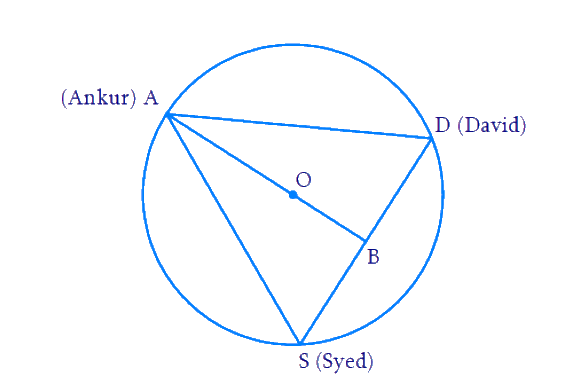Let \begin{align}{A, D, S}\end{align} denote the positions of Ankur, David and Syed respectively.

\begin{align}\Delta{ADS}\end{align} is an equilateral triangle since all the $$3$$ boys are on equidistant from one another.

Let $${B}$$ denote the mid-point of $${DS}$$ and hence $${AB}$$ is the median and perpendicular bisector of $${DS.}$$ Hence $$\Delta {.ABS}$$ is a right angled triangle with \begin {align} \angle {ABS}=90^{\circ} \end {align}

$$O$$ (centroid) divides the line $${AB}$$ in the ratio $$\text{2:1.}$$ So $${OA : OB = 2 : 1.}$$

\begin{align}\frac{{OA}}{{OB}} &= \frac{2}{1}\,\end{align}

\begin{align} \text{Since } OA &= 20 \; \rm {then}\\OB &=10\rm{m}\end{align}

\begin {align} {AB}&={OA}+{OB}\\ &=20+10 \\&=30 \, \rm{m}\qquad \ldots .(1) \end {align}

Let the side of equilateral triangle $$\Delta {ADS}$$ be $$2x.$$

\begin{align}{AD}&={DS} \\ &={SA}\\ &=2 x \ldots .(2) \end {align}

Since $${B}$$ is the mid-point of $${DS,}$$ we get

\begin {align}{BS}={BD}=x \dots(3)\end {align}

Applying Pythagoras theorem to $$\Delta {ABS,}$$ we get:

\begin {align}{AD}^{2}&={AB}^{2}+{BD}^{2} \\ {(2 x)^{2}}&={30^{2}+x^{2}} \\ 4 x^{2}&=900+x^{2}\\ 3 x^{2}&=900 \\ x^{2}&=\frac{900}{3}=300 \\ x&=\sqrt{300} \\ &=17.32 \, \rm {m}\end{align}

\begin {align}{AD}={DS}={SA}=2 x=34.64 \, \mathrm{m} \end {align}

Length of the string $$=$$ Distance between them

$$= AD$$ or $$DS$$ or $$SA = 34.64\,\rm m.$$

Instant doubt clearing with Cuemath Advanced Math Program